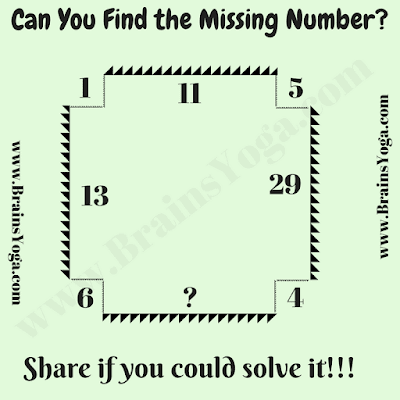If you are school going student, then this Fun Maths Puzzle will test your Mathematical and logical reasoning skills. In this Maths Puzzle, you challenge is to find the relationship among given number around the Rectangle and then find the value of missing number which replaces question mark. Can you take this challenge?Can you find the missing number?

The answer to this "Fun Maths Puzzle for Students", can be viewed by clicking on the button. Please do give your best try before looking at the answer.